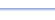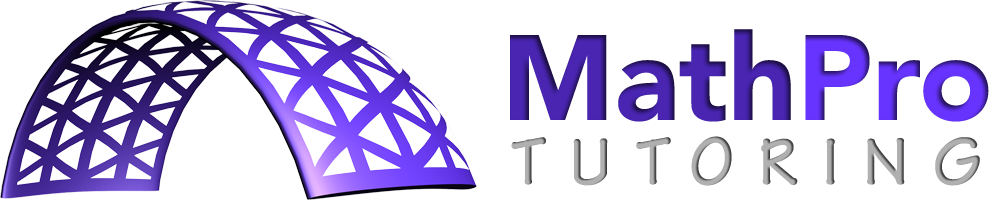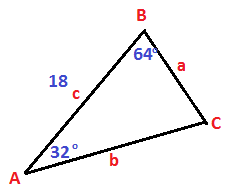Program: TRISOLVE

TRISOLVE

• Finds the missing sides and angles of a triangle, given various combinations of sides and angles.

Useful for:

• SAT
• ACT
• Geometry
• Precalculus
• Trigonometry
• Calculus

See full list of programs

Example

Q: Solve the triangle:
(Find the missing parts.)

Solution:

Run TRISOLVE.  How?
Select choice 4 for 'ASA'
because we are given
two angles and the
included side.

Enter:
ANGLE A = 32
ANGLE B = 64
SIDE c = 18

The program reports:
The first three numbers
on this list are the side
lengths: a, b, c.  The
last three numbers are
the angle measures:
A, B, C.

Interpretation:  The missing parts are:
ANGLE C = 84, SIDE a = 9.59, SIDE b = 16.27.

*Notes: 1) If your triangle has different letters, or the same letters in a different order, just rename the letters on your triangle to match the program.  2) In some instances, the program will report 'NO TRIANGLE', '1 TRIANGLE', or '2 TRIANGLES'.  Press ENTER to see the values.  Press ENTER again to see the second set of values for '2 TRIANGLES'.a -->
b -->
c ------------------->
A ------------------->
B ------------------->
C ------------------->